## Tuesday, 11 September 2012

### A Couple of Nice Extensions of the Median Properties

One of the common topics in HS Geometry is to expose students to the triangle concurrency theorems, and in particular the fact that the medians of a triangle intersect in a single point that breaks each median in a ratio of 2:1.

As student's proceed through the years of HS, there are a couple of nice extensions that I find pleasing to expose students to. Others may enjoy sharing them with their students as well.

Added 2015  I just noticed a nice post on a curiously sweet property of the  medians in a post from Alex Bogomolny at Cut-The-Knot.  I will just give a teaser of it here, and you can follow the link to Alex's complete proof.

Amazingly, the sum of the reciprocals of the odd numbered in-radii is equal to the sum of the reciprocals of the even numbered in-radii.
$\frac{1}{r_1} + \frac {1}{r_3} + \frac {1}{r_5} = \frac{1}{r_2} + \frac {1}{r_4} + \frac {1}{r_6}$

Commandino's Theorem.
If you use the tetrahedron as a generalization of the triangle into three-space, then the four lines from each vertex to the centroid of the opposite faces (where the medians of that triangular face intersect) then these four medial segments also share a common intersection. In this case the ratio of the two parts into which it divides the segment is 3:1. (I usually make the extra emphasis, 2:1 in 2-D; 3:1 in 3-D and suggest that if there was a closed 4-D simplex the medial segments from the vertex to the centroid of the tetrahedral bases would be divided 4:1 by a common intersection, although I haven't proven that.)

Commandino is remembered for translating many ancient texts. He presented this theorem in 1565 as Prop. 17 in his De Centro Gravitates Solidorum on Centers of Gravity.

I came across another interesting extension in a January 1951 "Mathematical Miscellanea" edited by Phillip S. Jones. The article contained a contribution by John Satterly of the University of Toronto on a type of cevian that he called "Nedians" which I wrote about a little over a year ago. [My personal choice, since the "med" root is for the middle, would have just been to call them n-dians, but I'm sure that would have had a cultural backlash.]

Instead of dividing each point on the triangles legs at the point 1/2 way between them, suppose we marked a point 1/3 (or 1/n) of the way.

Professor Satterly showed that the sum of the squares of the nedians would be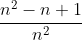times the sum of the squares of the sides of the original triangle. Notice that when n=2, this reduces to 3/4, the ratio given and proved for the medians in an earlier blog.

Professor Satterly also stated that the Nedian triangle will have an area of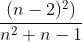times the area of ABC. Note that for the median, or 2-nedian, the area diminishes to zero since the three medians intersect in a single point.

Exploring these constructions a little more, I came up with a few more properties that were not in the article. For example, the perpendicular distance from the three vertices of the nedian triangle to any side of ABC will equal the altitude of ABC to that same side. [I call these the sub-altitudes (dotted red in the image below)]

The shortest sub-altitude will be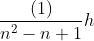, while the next longer one will be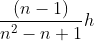and the longest will be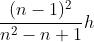.   In the example where the points are located 1/3 of the way along the side, the three sub-altitudes will be 1/7 h, 2/7 h, and 4/7 h.  This property applies to the altitude and sub-altitudes to each base.

I also observed that that each nedian is partitioned into three parts whose lengths, in order from the vertex to the opposite side, are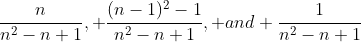.

For the 3-nedians in the image, for example, the nedian CF is partitioned so that CJ is 3/7of CF; JL is 3/7 of CF; and LF is 1/7 of LF. A similar partition holds for the other two 3-nedians AD and BE. In a 4-nedian, the partitions would be 4/14, 8/13, and 1/14.

Some or all of these are probably well within the range of a talented HS student.  I hope they are of interest to teachers and students.  Enjoy